Courses

# Test: AC Power Analysis

## 10 Questions MCQ Test Topicwise Question Bank for Electrical Engineering | Test: AC Power Analysis

Description
This mock test of Test: AC Power Analysis for Electrical Engineering (EE) helps you for every Electrical Engineering (EE) entrance exam. This contains 10 Multiple Choice Questions for Electrical Engineering (EE) Test: AC Power Analysis (mcq) to study with solutions a complete question bank. The solved questions answers in this Test: AC Power Analysis quiz give you a good mix of easy questions and tough questions. Electrical Engineering (EE) students definitely take this Test: AC Power Analysis exercise for a better result in the exam. You can find other Test: AC Power Analysis extra questions, long questions & short questions for Electrical Engineering (EE) on EduRev as well by searching above.
QUESTION: 1

### The value of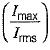for the waveform shown below is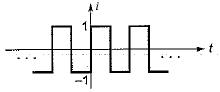Solution:

For the square wave shown in given figure,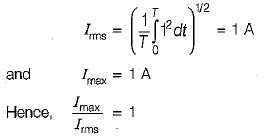QUESTION: 2

Solution:
QUESTION: 3

### The instantaneous power delivered to the 5 Ω resistor at t = 0 in the given circuit isSolution:

At t = 0, the given circuit is as shown below.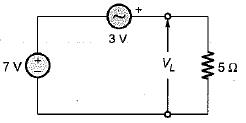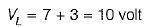∴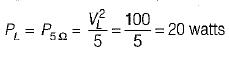QUESTION: 4

For a sinusoidal waveform, the ratio of average to rms value is

Solution:

For a sinusoidal waveform,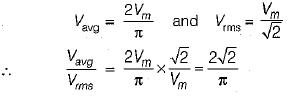QUESTION: 5

An impedance of (3 + j 4) Ω is connected in parallel with a resistance of 10 Ω. The ratio of power loss in these parallel circuits is

Solution: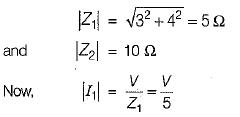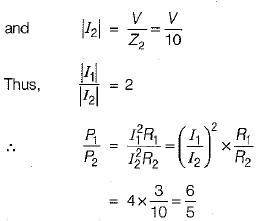QUESTION: 6

A 0.2 HP induction motor runs at an efficiency of 85%. If the operating power factor is 0.8 lag, the reactive power taken by the motor is

Solution: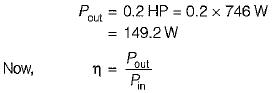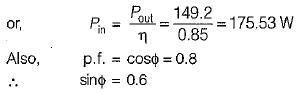∴ Reactive power taken by motor is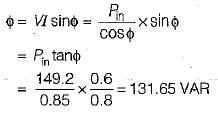QUESTION: 7

In an a.c. circuit, v = 100 sin (ωt + 30°) V, i = 5 sin(ωt- 30°) A. The apparant and reactive power in the circuit are respectively

Solution: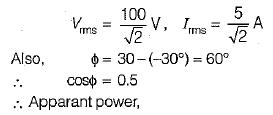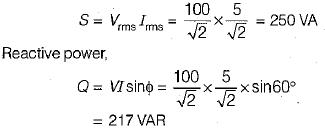QUESTION: 8

The inductive reactance in series with Z in the circuit shown below has a value of 25 Ω If the voltage drop a cross Z is 179 volts, the power dissipated in the circuit is 320 W.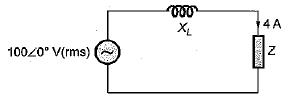The reactive part of Z is

Solution: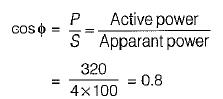Now, power dissipated,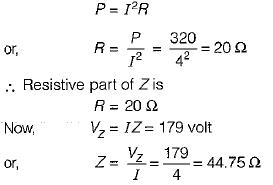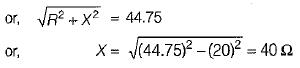QUESTION: 9

The values of current I1 and I2 in the circuit shown below are given by:

1A = (0.8 + j0.2) A and I2 = -(1 - j0.5) A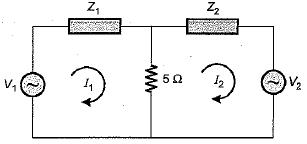The total power lost in the 5 Ω resistor is

Solution:

Power lost in the 5Ω resistor is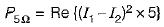(As current through 5 Ω resistor = I1 -I2)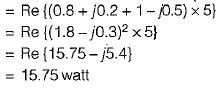QUESTION: 10

​The power factor of the circuit shown below is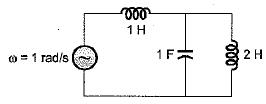Solution:

Given, ω = 1 rad/sec. So, the circuit can be redrawn as shown below.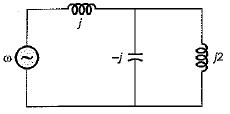Total impedance of the circuit is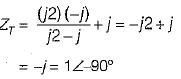∴ p.f. of the given circuit = cos90° = 0